Next: Regularization of the pairing Up: Examples Previous: Examples

## Self-consistent calculation for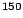Sn

As a first example we discuss here the results obtained for the semi magic isotopeSn (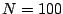and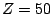) using the effective force SLy4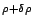. This nucleus is an interesting example since it has a rather small neutron Fermi energy (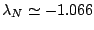MeV). Small neutron separation energy allows us to discuss possible effects of the integration box size as well as the choice of the boundary condition. If not explicitly stated otherwise, in all calculations the integration step is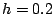fm for the box size of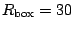fm, i.e., the number of integration points is 150+1. The global properties of the nucleus for Dirichlet or Neumann boundary condition are shown in table 5.

Table 5: Total binding energy, mean pairing gap, Fermi energy (all in MeV) and neutron rms radius (in fm) inSn, calculated for the force SLy4and two different boundary conditions: Dirichlet (vanishing wave functions at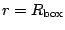) or Neumann (vanishing derivative of the wave functions at).
 Boundary condition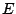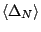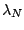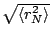Dirichlet -1131.863 -1.431 -1.067 5.264 Neumann -1131.862 -1.431 -1.067 5.264

In addition to the small neutron separation energy, one finds several single particle states with a significant occupation aroundwith different values of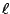and indeed different asymptotic bahaviour. The single particle neutron states in the vicinity of the Fermi energy are shown in table 6. It is gratifying to observe that despite the fact that the quasiparticle states are a little bit influenced by the change of the boundary conditions, the canonical states remain unchanged.

Table: Single particle neutron levels inSn using the effective force SLy4. Calculations have been made in a 30fm box with an integration step of 0.2fm.andare the quasiparticle energies and norms of the lower components while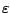is the canonical energy (54) and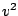is the occupation factor of the corresponding canonical state (55).
 Boundary condition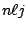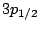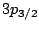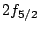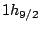Dirichlet(MeV) 0.926 0.937 1.330 1.556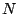0.221 0.577 0.254 0.438(MeV) 1.169 1.051 1.445 1.5720.251 0.619 0.264 0.438 Neumann(MeV) 0.919 0.932 1.328 1.5560.211 0.562 0.251 0.438(MeV) 1.168 1.051 1.445 1.5720.251 0.619 0.263 0.438

When a rather exotic nucleus is studied, it is important to check how well the results are converged with respect to the various approximations which are made in the numerical treatment of the HFB problem. First of all, we limit the calculation to a maximum number of partial wave given by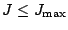, which has to by consistent with the mass of the nucleus. The convergence of the kinetic, pairing, and total energies are shown in the left part of Fig. 2. Between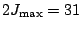and 37 the total energy difference is 1.3keV. The changes in the kinetic and pairing energies are a little bit bigger (7keV and 6keV, respectively); since these quantities are not at their extrema, they react more rapidly to a small change of the wave function.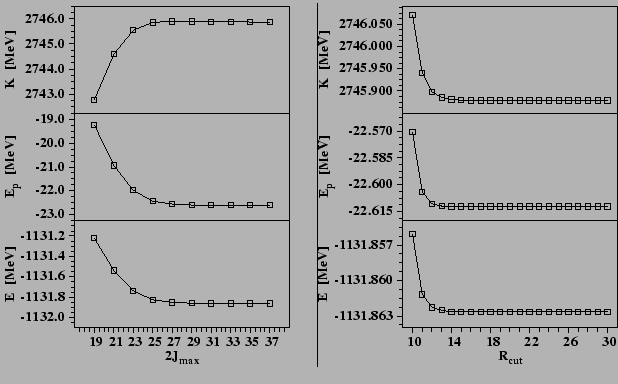As discussed in section 5.3, the introduction of the cut-off radius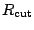in the pairing channel, Eq. (77), can improve the numerical stability of the iterative procedure when solving the HFB equation. The right part of Figure 2 displays the evolution of the kinetic, pairing, and total energies with the same parameters as in the left part of the Figure, except for the value of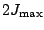which is fixed at 31. It can be clearly seen that beyond a rather small distance, the total energy and its components are not affected by the introduction of this cut-off. Specifically, for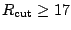fm the change in the total energy is less than 0.001keV.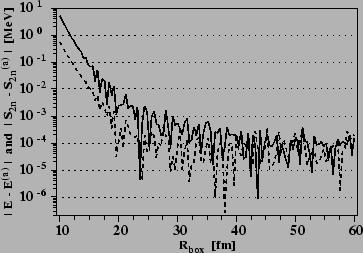Figure 3 shows the convergence of the total energyand two-neutron separation energy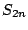as functions of the size of the box. Calculations have been made for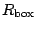ranging from 10 fm to 60 fm with a step of 0.4 fm. The cut-off equivalent energy of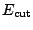=60MeV and the diffuseness of 1MeV have been used. In order to clearly exhibit the asymptotic trend of these quantities we have estimated their average asymptotic values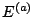and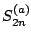. Since for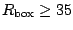fm the results do not show any significant evolution, these values are estimated by taking averages for 35 fm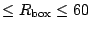fm, which gives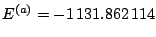MeV and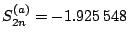MeV. Here, the last significant digit corresponds to 0.001keV, which is the accuracy required to stop the iterations when we solve the HFB equations for any given value of. The fluctuations around the average values result from the individual continuum states entering into the cut-off window with increasing. We see that for relatively small boxes (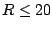fm) the two-neutron separation energy is an order of magnitude more stable than the total binding energy of the nucleus. For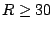fm, the two quantities reach their asymptotic values with a random dispersion of about 0.5 keV. The value of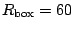fm is obviously unnecessarily large but it represents a good test of the stability of the integration procedure. From these results we conclude that the solution of the HFB equations with the box boundary conditions and the energy cut-off is precise up to about 1keV.

A typical asymptotic behaviour of the particle and pairing densities for neutrons is shown in Fig. 4 for two different choices of the boundary condition. The impact of the boundary conditions only shows up within about 5fm near the box edge. It is small enough to have no significant effect on the calculated observables.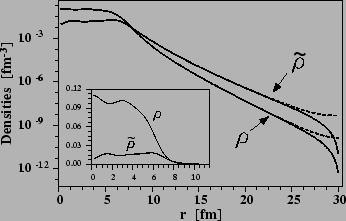Neutron particle and pairing densities for different sizes of the box are shown in Fig. 5. The box radius of 10fm is obviously too small and it has only been included to show the effect of a very small box on the densities. For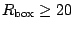fm, no differences can be seen in the linear scale. An interesting fact is that despite the cut that has been applied for the pairing field at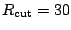fm, see Sec. 5.3, no consequences can be observed on the densities in the asymptotic region.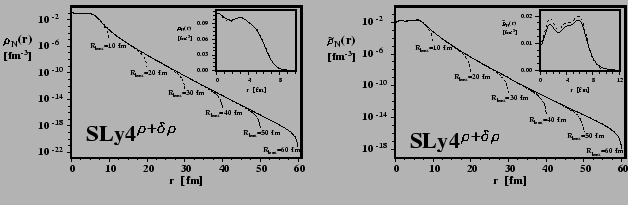Next: Regularization of the pairing Up: Examples Previous: Examples
Jacek Dobaczewski 2005-01-23